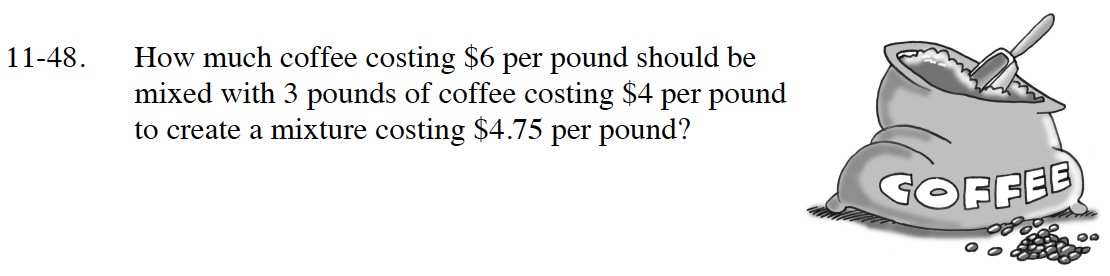Home > CC4 > Chapter 11 > Lesson 11.2.1 > Problem11-48

11-48.
1. How much coffee costing $6 per pound should be mixed with 3 pounds of coffee costing$4 per pound to create a mixture costing $4.75 per pound? Homework Help ✎Three pounds of$4/lb coffee is worth $12. Let x = the number of pounds of$6/lb coffee.
Then 6x = the cost of that much coffee. Put the total cost of the coffee (DOLLARS) over (PER) the total number of pounds (POUND) equal to \$4.75 per pound.
Then solve for x.

$\frac{\left(12+6\textit{x}\right)}{3+\textit{x}}=4.75$